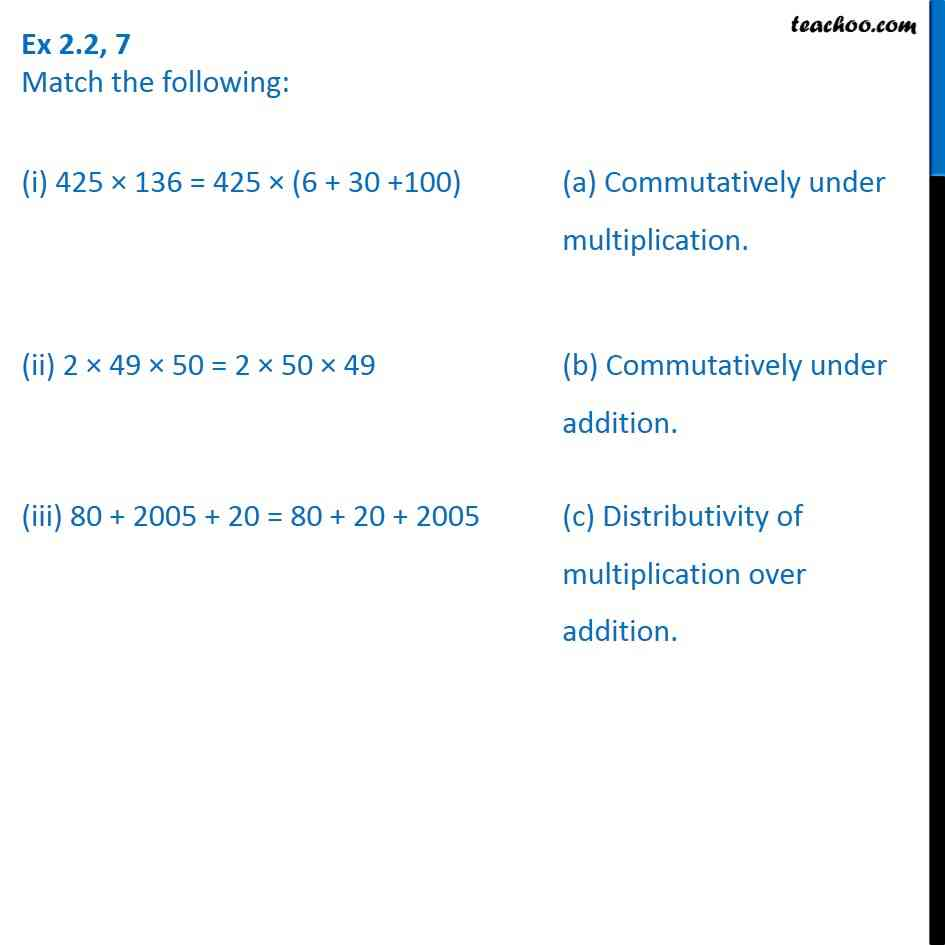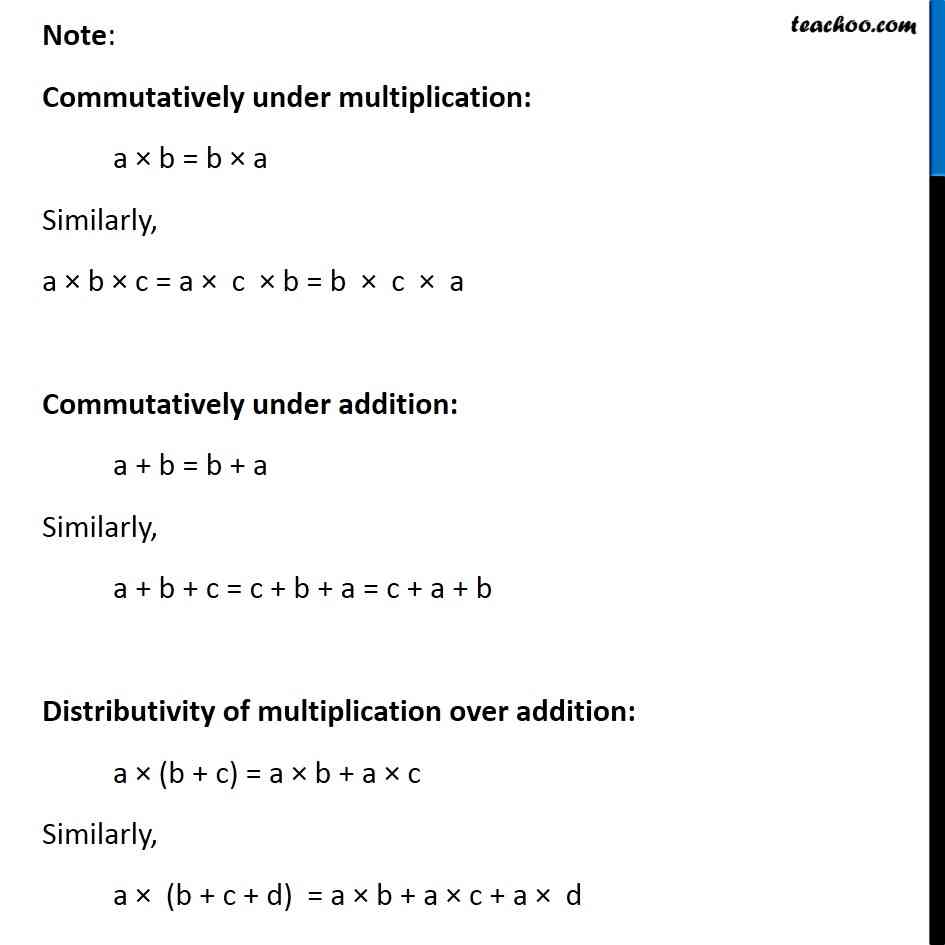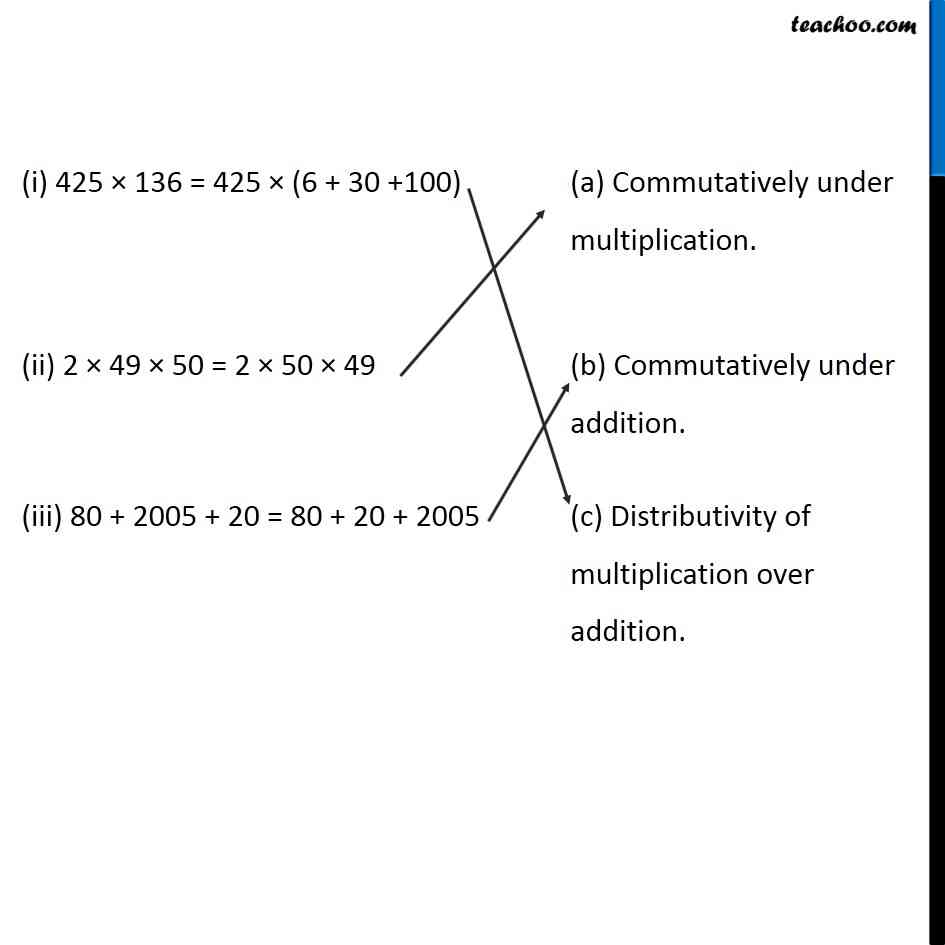1. Chapter 2 Class 6 Whole Numbers
2. Concept wise
3. Distributivity

Transcript

Ex 2.2, 7 Match the following: (i) 425 × 136 = 425 × (6 + 30 + 100) (ii) 2 × 49 × 50 = 2 × 50 × 49 (iii) 80 + 2005 + 20 = 80 + 20 + 2005 (a) Commutatively under multiplication (b) Commutatitively under addition (c) Distributivity of multiplication over addition Note: Commutatively under multiplication: a × b = b × a Similarly, a × b × c = a × c × b = b × c × a Commutatively under addition: a + b = b + a Similarly, a + b + c = c + b + a = c + a + b Distributivity of multiplication over addition: a × (b + c) = a × b + a × c Similarly, a × (b + c + d) = a × b + a × c + a × d